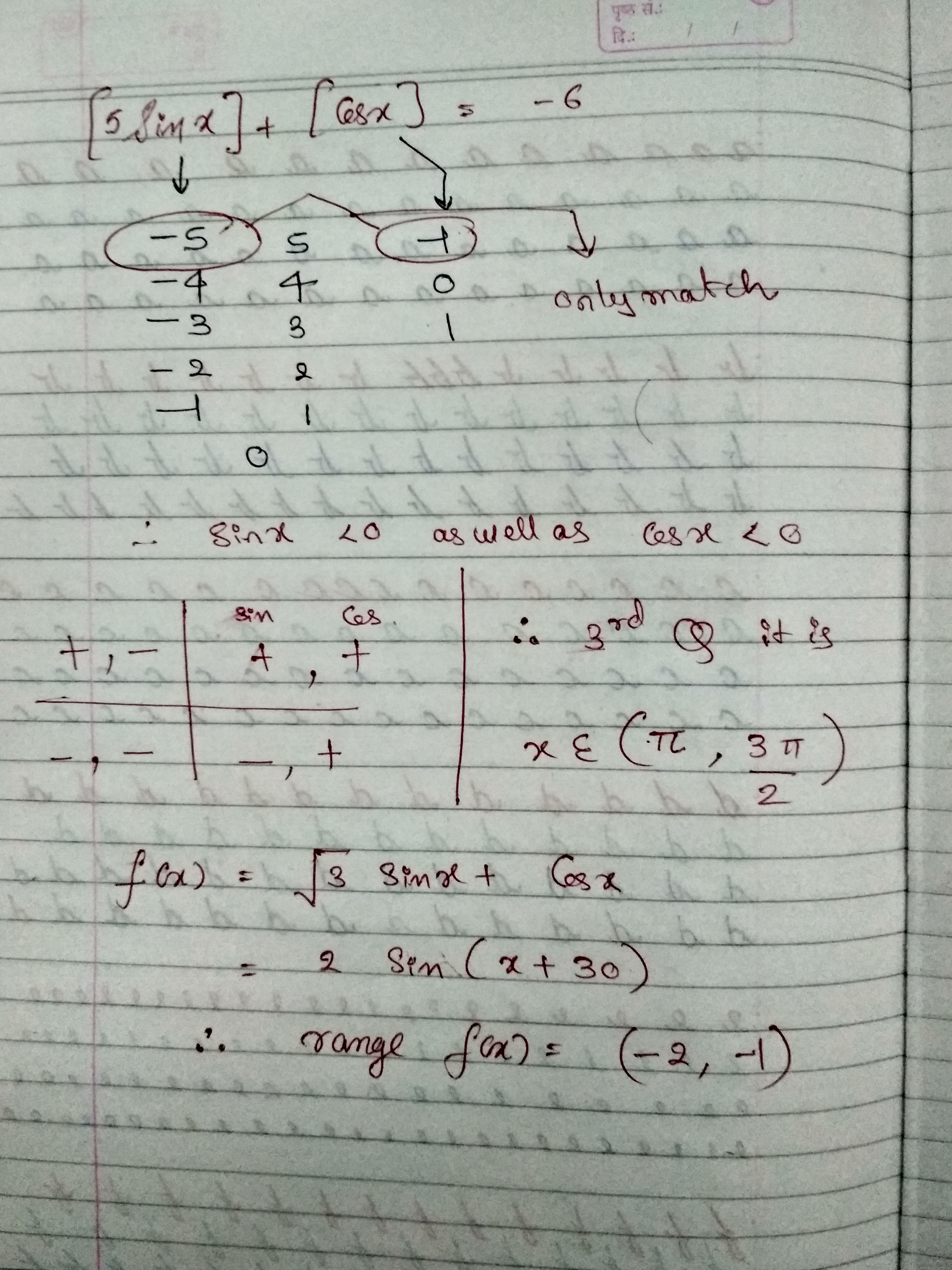×#### Thank you for registering.

One of our academic counsellors will contact you within 1 working day.

Click to Chat

1800-1023-196

+91-120-4616500

CART 0

• 0

MY CART (5)

Use Coupon: CART20 and get 20% off on all online Study Material

ITEM
DETAILS
MRP
DISCOUNT
FINAL PRICE
Total Price: Rs.

There are no items in this cart.
Continue Shopping
```
Question number 19: if [5sinx]+[cosx]+6=0, then range of f(x)=3^1/2 cosx + sinx corresponding to solution set of the given equation is: a) [-2,-1) b)(-(3(3^1/2)+2)/5, -1) c) [-2,-3^1/2) d)( -(3 (3^1/2) + 4)/5 , 1)

```
one year ago

```							The value of [5Sinx] + [cosx] must be equal to -6 5Sinx must be equal to -5 and [cosx] must be equal to -1 -1and  -1 Find the intersection of both these solutions , and then Put this vaue in the f(x) which you want to find the range .
```
one year ago
```							You need to find the intersection of solution of -1 and -1Take intersection of both the equation and put the value in f(x)
```
one year agoSaurabh Koranglekar
10233 Points
``````
one year ago
```							I think there is problem in software , the thing i am typing is not uploading , well You need to find the intersection of solution of -1 less than or equa to sinx less than or equal to -4/5 and -1 less than or equal to cosx less than or equal to 0 .
```
one year ago
Think You Can Provide A Better Answer ?

## Other Related Questions on Algebra

View all Questions »### Course Features

• 731 Video Lectures
• Revision Notes
• Previous Year Papers
• Mind Map
• Study Planner
• NCERT Solutions
• Discussion Forum
• Test paper with Video Solution### Course Features

• 101 Video Lectures
• Revision Notes
• Test paper with Video Solution
• Mind Map
• Study Planner
• NCERT Solutions
• Discussion Forum
• Previous Year Exam Questions